Vibrations and Waves - Lesson 2 - Properties of a Wave

# The Anatomy of a Wave

A transverse wave is a wave in which the particles of the medium are displaced in a direction perpendicular to the direction of energy transport. A transverse wave can be created in a rope if the rope is stretched out horizontally and the end is vibrated back-and-forth in a vertical direction. If a snapshot of such a transverse wave could be taken so as to freeze the shape of the rope in time, then it would look like the following diagram.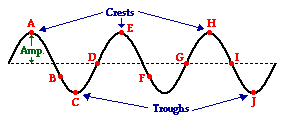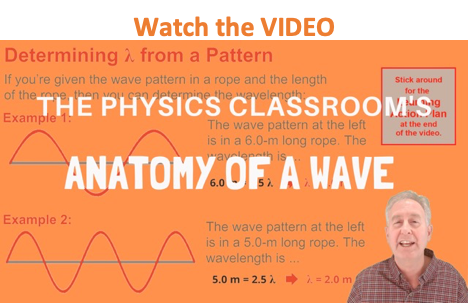The dashed line drawn through the center of the diagram represents the equilibrium or rest position of the string. This is the position that the string would assume if there were no disturbance moving through it. Once a disturbance is introduced into the string, the particles of the string begin to vibrate upwards and downwards. At any given moment in time, a particle on the medium could be above or below the rest position. Points A, E and H on the diagram represent the crests of this wave. The crest of a wave is the point on the medium that exhibits the maximum amount of positive or upward displacement from the rest position. Points C and J on the diagram represent the troughs of this wave. The trough of a wave is the point on the medium that exhibits the maximum amount of negative or downward displacement from the rest position.

The wave shown above can be described by a variety of properties. One such property is amplitude. The amplitude of a wave refers to the maximum amount of displacement of a particle on the medium from its rest position. In a sense, the amplitude is the distance from rest to crest. Similarly, the amplitude can be measured from the rest position to the trough position. In the diagram above, the amplitude could be measured as the distance of a line segment that is perpendicular to the rest position and extends vertically upward from the rest position to point A.

The wavelength is another property of a wave that is portrayed in the diagram above. The wavelength of a wave is simply the length of one complete wave cycle. If you were to trace your finger across the wave in the diagram above, you would notice that your finger repeats its path. A wave is a repeating pattern. It repeats itself in a periodic and regular fashion over both time and space. And the length of one such spatial repetition (known as a wave cycle) is the wavelength. The wavelength can be measured as the distance from crest to crest or from trough to trough. In fact, the wavelength of a wave can be measured as the distance from a point on a wave to the corresponding point on the next cycle of the wave. In the diagram above, the wavelength is the horizontal distance from A to E, or the horizontal distance from B to F, or the horizontal distance from D to G, or the horizontal distance from E to H. Any one of these distance measurements would suffice in determining the wavelength of this wave.

A longitudinal wave is a wave in which the particles of the medium are displaced in a direction parallel to the direction of energy transport. A longitudinal wave can be created in a slinky if the slinky is stretched out horizontally and the end coil is vibrated back-and-forth in a horizontal direction. If a snapshot of such a longitudinal wave could be taken so as to freeze the shape of the slinky in time, then it would look like the following diagram.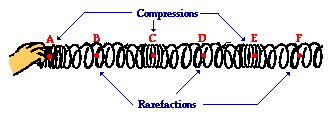Because the coils of the slinky are vibrating longitudinally, there are regions where they become pressed together and other regions where they are spread apart. A region where the coils are pressed together in a small amount of space is known as a compression. A compression is a point on a medium through which a longitudinal wave is traveling that has the maximum density. A region where the coils are spread apart, thus maximizing the distance between coils, is known as a rarefaction. A rarefaction is a point on a medium through which a longitudinal wave is traveling that has the minimum density. Points A, C and E on the diagram above represent compressions and points B, D, and F represent rarefactions. While a transverse wave has an alternating pattern of crests and troughs, a longitudinal wave has an alternating pattern of compressions and rarefactions.

As discussed above, the wavelength of a wave is the length of one complete cycle of a wave. For a transverse wave, the wavelength is determined by measuring from crest to crest. A longitudinal wave does not have crest; so how can its wavelength be determined? The wavelength can always be determined by measuring the distance between any two corresponding points on adjacent waves. In the case of a longitudinal wave, a wavelength measurement is made by measuring the distance from a compression to the next compression or from a rarefaction to the next rarefaction. On the diagram above, the distance from point A to point C or from point B to point D would be representative of the wavelength.

### We Would Like to Suggest ...Why just read about it and when you could be interacting with it? Interact - that's exactly what you do when you use one of The Physics Classroom's Interactives. We would like to suggest that you combine the reading of this page with the use of our Simple Wave Simulator. You can find it in the Physics Interactives section of our website. The Simple Wave Simulator provides the learner an environment to explore the distinction between longitudinal and transverse waves, the wavelength-frequency-period relationship, sound waves as pressure waves, and much more.

Consider the diagram below in order to answer questions #1-2.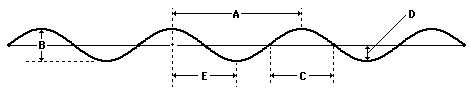1. The wavelength of the wave in the diagram above is given by letter ______.

2. The amplitude of the wave in the diagram above is given by letter _____.

3. Indicate the interval that represents one full wavelength.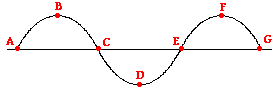a. A to C

b. B to D

c. A to G

d. C to G

Next Section: